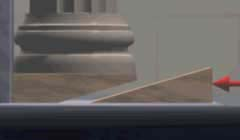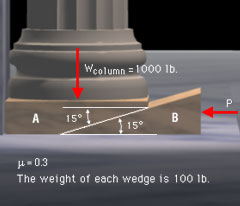Ch 9. Friction Multimedia Engineering Statics Friction I Friction II
 Chapter 1. Basics 2. Vectors 3. Forces 4. Moments 5. Rigid Bodies 6. Structures 7. Centroids/Inertia 8. Internal Loads 9. Friction 10. Work & Energy Appendix Basic Math Units Sections Search eBooks Dynamics Fluids Math Mechanics Statics Thermodynamics Author(s): Kurt Gramoll ©Kurt GramollSTATICS - CASE STUDY IntroductionProblem GraphicWedge King Ignoramus Maximus has established a lowest bid construction policy for the building of new temples. As a result, a column erected in the temple of Apollo was several inches too short. In order to raise the column, engineers will use two small marble wedges. What is known: The weight of the column is 1000 lb. The weight of each wedge is 100 lb.     The coefficient of friction is 0.3. The angle of the wedge is 15°. The column and top wedge are constrained against movement to the left. QuestionsForce Diagram What is the smallest horizontally applied force that will start the column moving upward? If the applied force and lateral constraints are removed, will the system remain in equilibrium? Approach Determine the forces acting on each wedge. Use any special properties of friction that could be helpful to simplify the problem.

Practice Homework and Test problems now available in the 'Eng Statics' mobile app
Includes over 500 problems with complete detailed solutions.
Available now at the Google Play Store and Apple App Store.